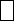Mathematics
Easy

Question

# Find the missing digit in the equation.78 – 36 =2

## 4563Hint:

## The correct answer is: 4

### 78 – 36 = 42The missing digit is 4.

Just use simple subtraction to solve this problem.

### Related Questions to study#### With Turito Foundation.#### Get an Expert Advice From Turito.